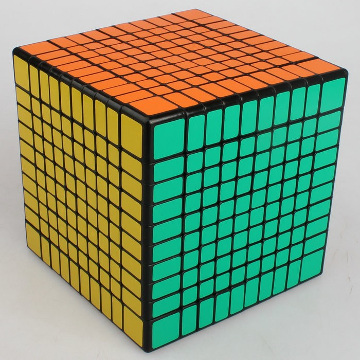# Inner Cube Unaffected

Probability Level 2On a $3\times3\times3$ cube, all but one $1\times1\times1$ are visible.

How many $1\times1\times1$ cubes are visible on the surface of a $10\times10\times10$ cube?

×